Miscellaneous

Chapter 13 Class 11 Limits and Derivatives
Serial order wise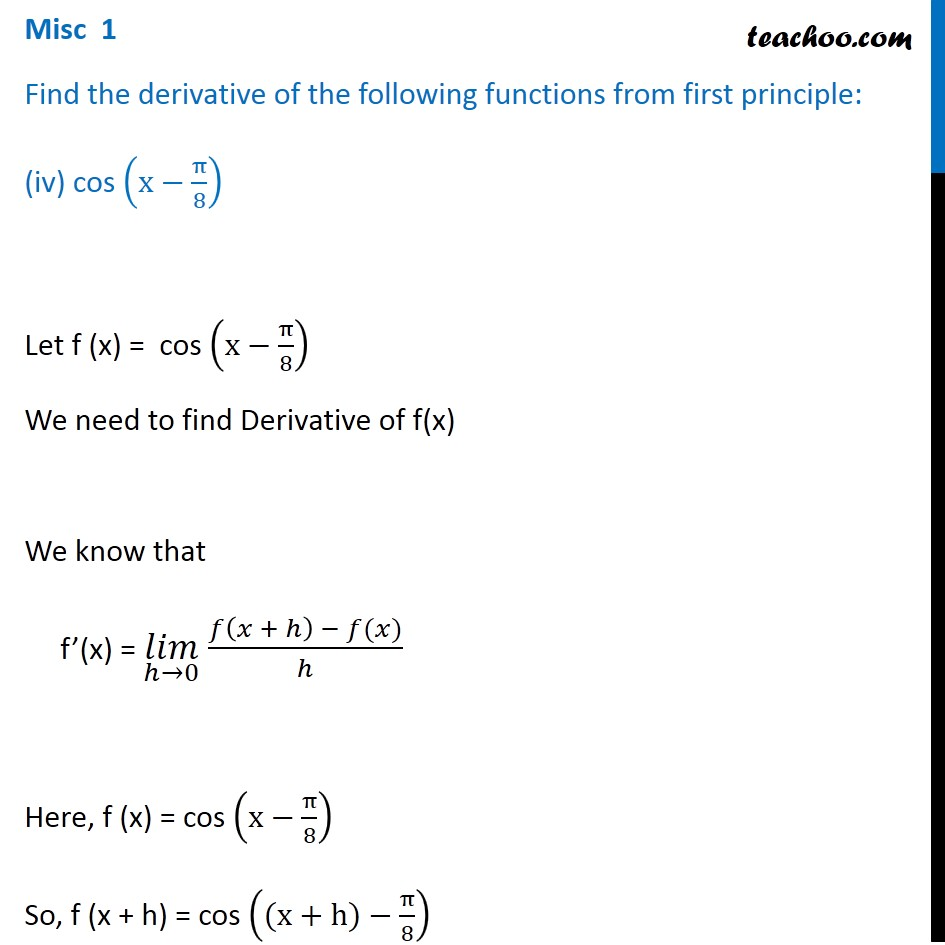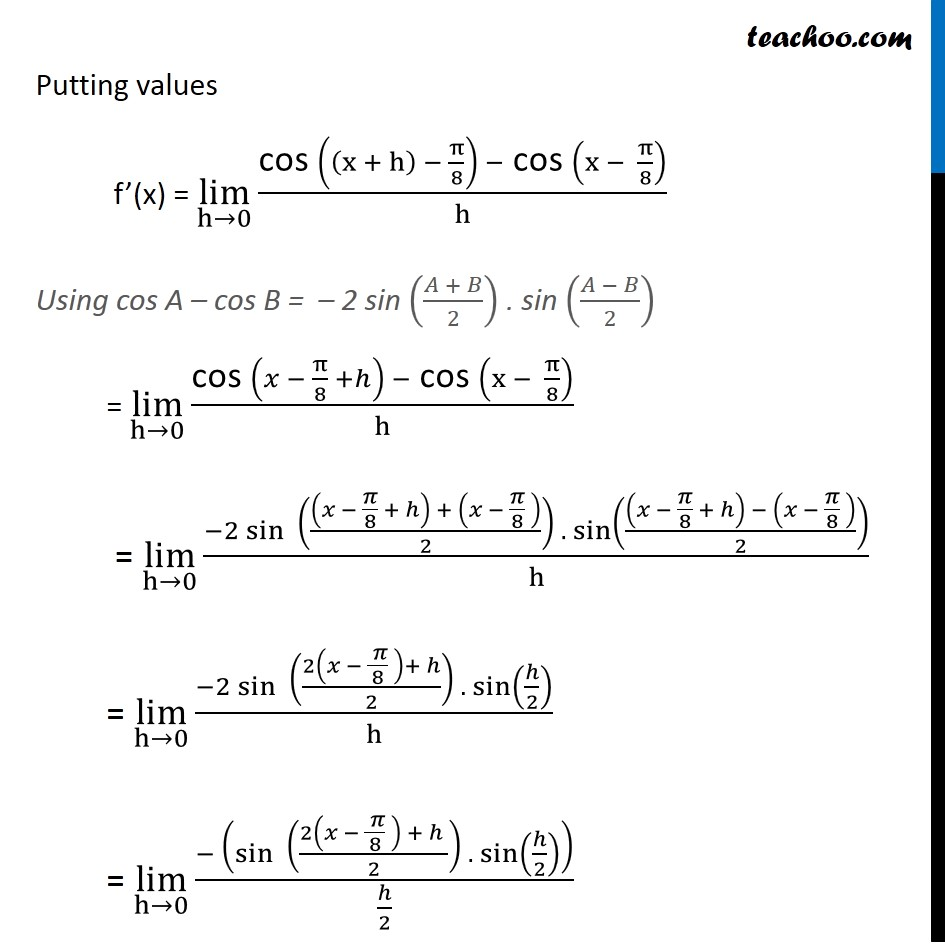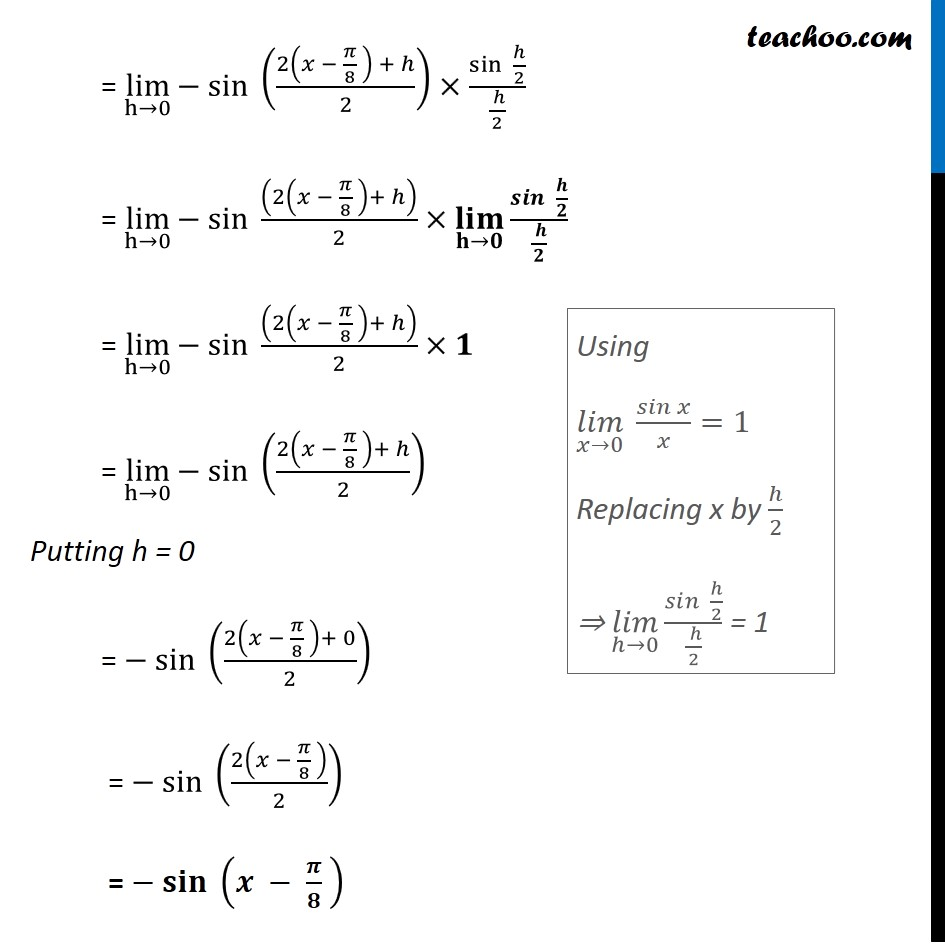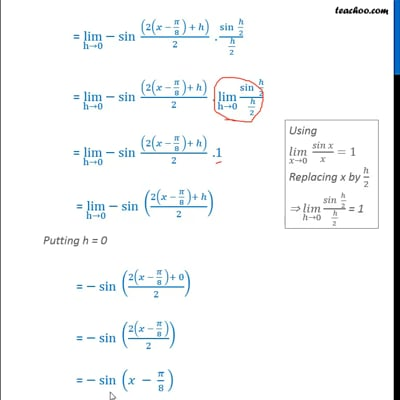This video is only available for Teachoo black users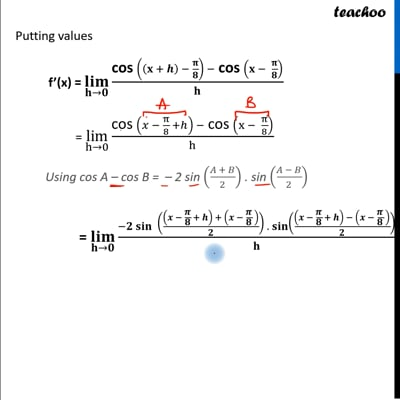This video is only available for Teachoo black users

Get live Maths 1-on-1 Classs - Class 6 to 12

### Transcript

Misc 1 Find the derivative of the following functions from first principle: (iv) cos (x−π/8) Let f (x) = cos (x−π/8) We need to find Derivative of f(x) We know that f’(x) = (𝑙𝑖𝑚)┬(ℎ→0) 𝑓⁡〖(𝑥 + ℎ) − 𝑓(𝑥)〗/ℎ Here, f (x) = cos (x−π/8) So, f (x + h) = cos ((x+h)−π/8) Putting values f’(x) = lim┬(h→0)⁡〖("cos " ((x + h) − π/8) −" cos " (x − π/8))/h〗 Using cos A – cos B = – 2 sin ((𝐴 + 𝐵)/2) . sin ((𝐴 − 𝐵)/2) = lim┬(h→0)⁡〖("cos " (𝑥 − π/8 +ℎ) −" cos " (x − π/8))/h〗 = lim┬(h→0)⁡〖〖−2 sin〗⁡〖 (((𝑥 − 𝜋/8 + ℎ) + (𝑥 − 𝜋/8 ))/2) . sin⁡(((𝑥 − 𝜋/8 + ℎ) − (𝑥 − 𝜋/8 ))/2) 〗/h〗 = lim┬(h→0)⁡〖(−2 sin⁡〖 ((2(𝑥 − ( 𝜋)/8 )+ ℎ)/2) . sin⁡(ℎ/2) 〗)/h〗 = lim┬(h→0)⁡〖(− (sin⁡〖 ((2(𝑥 − ( 𝜋)/8 ) + ℎ )/2) . sin⁡(ℎ/2) 〗 ))/(ℎ/2)〗 = lim┬(h→0)⁡〖〖− sin 〗⁡((2(𝑥 − 𝜋/8 ) + ℎ)/2)×sin⁡〖 ℎ/2〗/(( ℎ)/2)〗 = lim┬(h→0)⁡〖〖− sin 〗⁡〖((2(𝑥 − 𝜋/8 )+ ℎ))/2〗×(𝐥𝐢𝐦)┬(𝐡→𝟎) 𝒔𝒊𝒏⁡〖 𝒉/𝟐〗/(( 𝒉)/𝟐)〗 = lim┬(h→0)⁡〖〖− sin 〗⁡〖((2(𝑥 − 𝜋/8 )+ ℎ))/2〗×𝟏〗 = lim┬(h→0)⁡〖〖− sin 〗⁡((2(𝑥 − 𝜋/8 )+ ℎ)/2) 〗 Putting h = 0 = 〖− sin 〗⁡((2(𝑥 − 𝜋/8 )+ 0)/2) = 〖− sin 〗⁡(2(𝑥 − 𝜋/8 )/2) = 〖− 𝐬𝐢𝐧 〗⁡(𝒙 − 𝝅/𝟖 ) Using (𝑙𝑖𝑚)┬(𝑥→0)⁡〖 𝑠𝑖𝑛⁡𝑥/𝑥〗=1 Replacing x by ℎ/2 ⇒ (𝑙𝑖𝑚)┬(ℎ→0) 𝑠𝑖𝑛⁡〖 ℎ/2〗/(( ℎ)/2) = 1